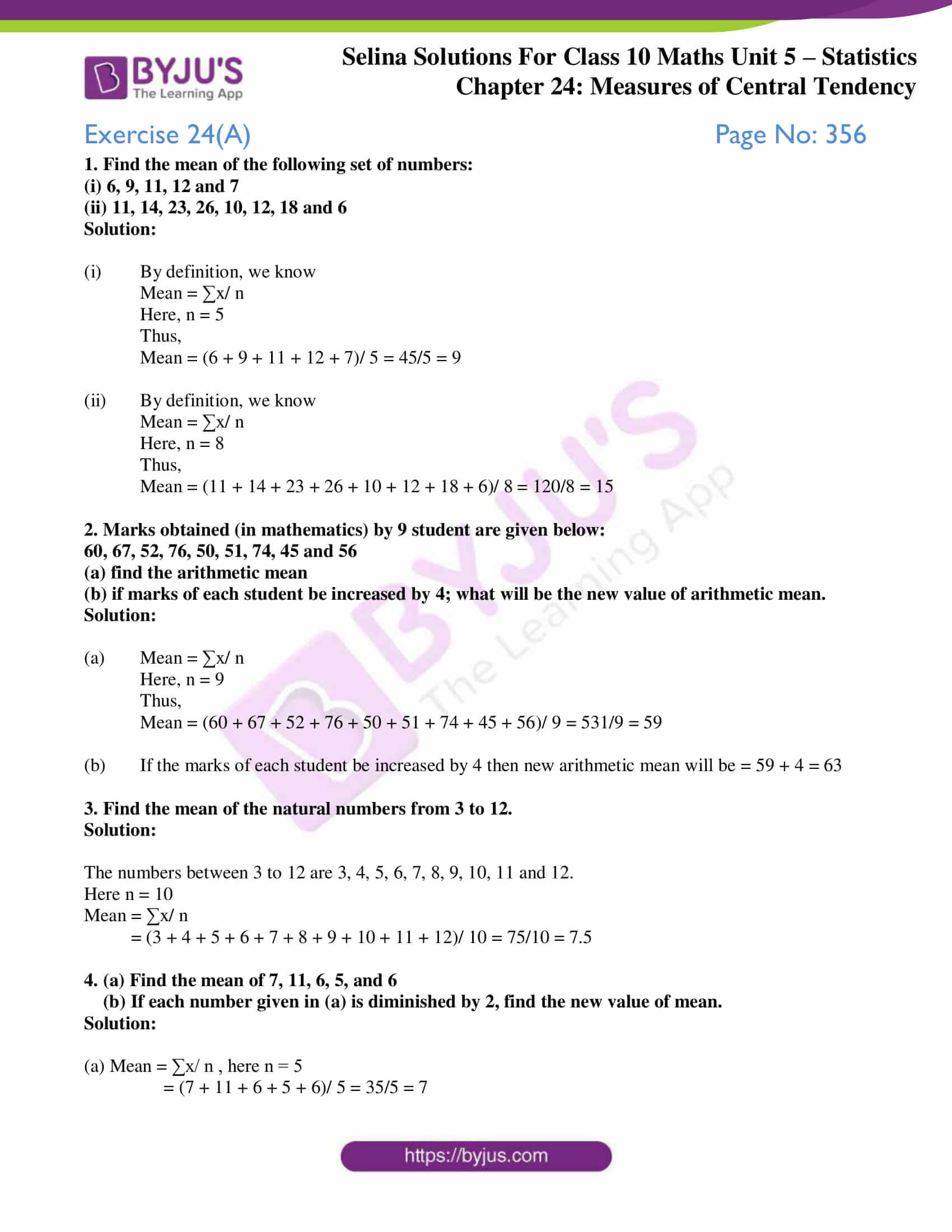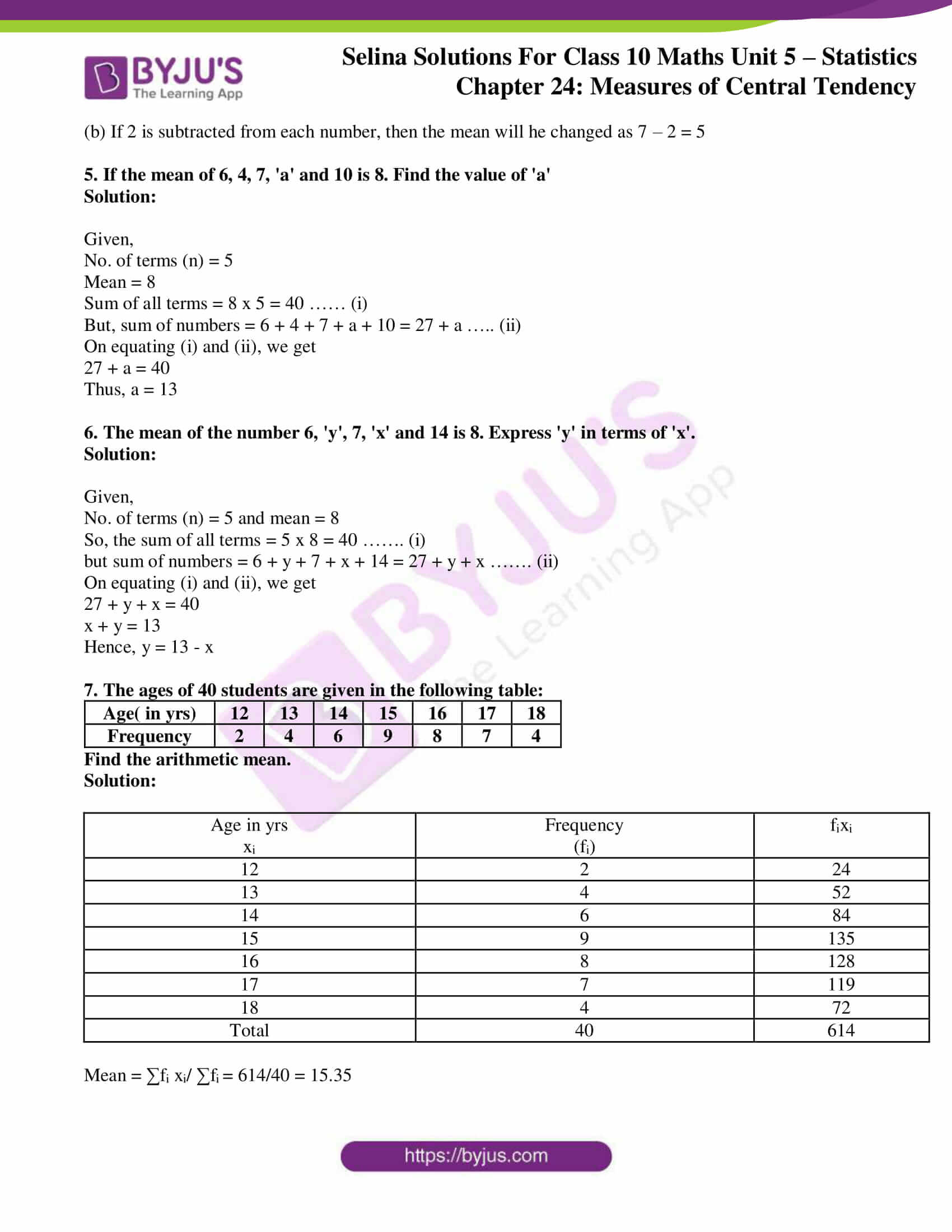# Selina Solutions Concise Maths Class 10 Chapter 24 Measures of Central Tendency Exercise 24(A)

The arithmetic mean or average of tabulated data can be obtained in three methods; 1. Direct method 2. Short-cut method and 3. Step-deviation method. Hence, this exercise deals with problems on calculating the arithmetic mean in any of these methods. The Selina Solutions for Class 10 Maths is a one-stop solution to all doubts and clarifications in solving problems of this chapter and others. The solutions of this exercise are available in the Concise Selina Solutions for Class 10 Maths Chapter 24 Measures of Central Tendency Exercise 24(A) PDF, which can be accessed from the link below.

## Selina Solutions Concise Maths Class 10 Chapter 24 Measures of Central Tendency Exercise 24(A) Download PDF### Access other exercises of Selina Solutions Concise Maths Class 10 Chapter 24 Measures of Central Tendency

Exercise 24(B) Solutions

Exercise 24(C) Solutions

Exercise 24(D) Solutions

Exercise 24(E) Solutions

### Access Selina Solutions Concise Maths Class 10 Chapter 24 Measures of Central Tendency Exercise 24(A)

1. Find the mean of the following set of numbers:

(i) 6, 9, 11, 12 and 7

(ii) 11, 14, 23, 26, 10, 12, 18 and 6

Solution:

(i) By definition, we know

Mean = ∑x/ n

Here, n = 5

Thus,

Mean = (6 + 9 + 11 + 12 + 7)/ 5 = 45/5 = 9

(ii) By definition, we know

Mean = ∑x/ n

Here, n = 8

Thus,

Mean = (11 + 14 + 23 + 26 + 10 + 12 + 18 + 6)/ 8 = 120/8 = 15

2. Marks obtained (in mathematics) by 9 student are given below:

60, 67, 52, 76, 50, 51, 74, 45 and 56

(a) find the arithmetic mean

(b) if marks of each student be increased by 4; what will be the new value of arithmetic mean.

Solution:

(a) Mean = ∑x/ n

Here, n = 9

Thus,

Mean = (60 + 67 + 52 + 76 + 50 + 51 + 74 + 45 + 56)/ 9 = 531/9 = 59

(b) If the marks of each student be increased by 4 then new arithmetic mean will be = 59 + 4 = 63

3. Find the mean of the natural numbers from 3 to 12.

Solution:

The numbers between 3 to 12 are 3, 4, 5, 6, 7, 8, 9, 10, 11 and 12.

Here n = 10

Mean = ∑x/ n

= (3 + 4 + 5 + 6 + 7 + 8 + 9 + 10 + 11 + 12)/ 10 = 75/10 = 7.5

4. (a) Find the mean of 7, 11, 6, 5, and 6

(b) If each number given in (a) is diminished by 2, find the new value of mean.

Solution:

(a) Mean = ∑x/ n , here n = 5

= (7 + 11 + 6 + 5 + 6)/ 5 = 35/5 = 7

(b) If 2 is subtracted from each number, then the mean will he changed as 7 – 2 = 5

5. If the mean of 6, 4, 7, ‘a’ and 10 is 8. Find the value of ‘a’

Solution:

Given,

No. of terms (n) = 5

Mean = 8

Sum of all terms = 8 x 5 = 40 …… (i)

But, sum of numbers = 6 + 4 + 7 + a + 10 = 27 + a ….. (ii)

On equating (i) and (ii), we get

27 + a = 40

Thus, a = 13

6. The mean of the number 6, ‘y’, 7, ‘x’ and 14 is 8. Express ‘y’ in terms of ‘x’.

Solution:

Given,

No. of terms (n) = 5 and mean = 8

So, the sum of all terms = 5 x 8 = 40 ……. (i)

but sum of numbers = 6 + y + 7 + x + 14 = 27 + y + x ……. (ii)

On equating (i) and (ii), we get

27 + y + x = 40

x + y = 13

Hence, y = 13 – x

7. The ages of 40 students are given in the following table:

 Age( in yrs) 12 13 14 15 16 17 18 Frequency 2 4 6 9 8 7 4

Find the arithmetic mean.

Solution:

 Age in yrs xi Frequency (fi) fixi 12 2 24 13 4 52 14 6 84 15 9 135 16 8 128 17 7 119 18 4 72 Total 40 614

Mean = ∑fi xi/ ∑fi = 614/40 = 15.35